Next: Level crossings and the Up: Single-particle structures at N=Z=16 Previous: Coulomb effects and isospin

## Classification of SD bands

For the conserved parity,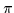=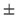, and signature, r=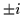, quantum numbers, the space of single particle states is separated into four parity/signature blocks,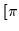,-ir]=(++,+-,-+,-). By constructing a particle-hole excitation we necessarily arrive at a rearrangement among the four blocks of levels - one class of rearrangements always leading to the occupation of all the lowest levels within each block. It turns out that such states form a majority among the low-lying bands studied here. Supposing that the lowest states in each of the blocks are occupied, one may describe in the standard way the many-particle configurations by giving the numbers of states occupied in each block. In this notation, the SD 32S magic configuration is given by the (4,4,4,4) occupation numbers, both for neutrons and protons, while the ground-state configuration reads (5,5,3,3).

All configurations that are examined below are built by exciting particles from the four levels below, to the four levels above the neutron and/or proton Fermi energies at the SD shape. The remaining orbitals below the Fermi levels will always be occupied. Therefore, the single-particle neutron or proton active spaces are composed of 8 orbitals (4 intruders and 4 non-intruders) that contain 4 particles. This leads to C84=70 possible many-body SD configurations for neutrons and C84=70 SD configurations for protons. The fact that among the bands studied in this article always the lowest states in each parity/signature block are occupied (other cases, where necessary, will be explicitly mentioned) reduces these numbers from 70 to 19 neutron or proton configurations necessary to control the low-energy rotational bands constructed within the discussed active spaces.

Further, we use the observation that for both intruder states, 1/2 and 3/2, the r=+i signature partners are always below the r=-i partners (for all deformations and rotational frequencies). Hence, the intruder orbitals should preferably be occupied in that order of increasing energy, i.e., when one particle occupies the negative-parity orbitals, it will occupy the 1/2(r=+i) orbital, when two - they will occupy the 1/2(r=+i) and 1/2(r=-i) orbitals, and when three - they will occupy the 1/2(r=+i), 1/2(r=-i), and 3/2(r=+i) orbitals, etc. This rule reduces the number of available configurations from 19 to 9. Finally, we reject two more configurations, as described below, and we are left with 7 configurations to be considered for neutrons and for protons. Although such a preselection of configurations may appear to be quite arbitrary, it is in fact based on the requirement that one wants to end up with a restricted set of low-energy configurations only.

Figure 3 shows schematically the single-particle orbitals (top), as well as all the considered here particle-hole configurations (bottom). The same diagram is valid both for neutrons and protons. The four intruder-states that are close to the Fermi energies are all characterized by the principal HO quantum number N0=3. Following the well-established notation, we denote the neutron or proton intruder occupations by the symbol 3n/p, where n or p are the numbers of the occupied neutron and proton intruder states, respectively.

As illustrated in the figure, for the 30 or 34 configurations, there are four or none particles, respectively, in the positive-parity states, and hence, in our predefined phase space, these configurations are unique. For the 32 configuration, two particles occupy the positive-parity states, and we restrict our considerations to only one (out of three) occupation variant, namely, we require both particles to occupy the two signatures of the lower orbital 1/2. Hence, in the following, symbol 32 pertains to this particular configuration. Finally, if one or three intruder states are occupied, i.e., in the 31 or 33 configurations, there are accordingly, three particles or one particle in the positive-parity orbitals, and here an additional label is necessary. We distinguish the corresponding configurations by introducing subscripts + or -, i.e., by using symbols 31+, 31-, 33+, 33-. The subscripts correspond, (i) in the 33 configurations, to the signature of the occupied 1/2(r=) orbital, and (i) in the 31 configurations, to the signature of the occupied 5/2(r=) orbital. Whenever symbols 31 or 33 without the subscripts are used, they pertain to both such configurations.

After having preselected the 7 neutron and proton configurations, we have at our disposal 49 configurations of the whole nucleus, which we denote by 3n3p, and when necessary supplement by the signature subscripts + or -, as described above. For example, the magic SD configuration of 32S is denoted by 3232, and the ground-state configuration reads 3030.

A manifest symmetry between neutrons and protons implies a manifest symmetry between the corresponding rotational bands. We have verified that those bands which are mirror images obtained from one another by replacing the neutrons by the protons and vice versa lead to almost identical results. However, because of the larger spatial extensions of intruder orbitals as compared to positive-parity orbitals, the (very small) Coulomb shifts will always slightly bring down the p>n configurations below those with p<n. Consequently, in the following we consider only the 3n3p configurations for p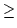n. Introducing these last arguments into our selection scheme, we end up with 30 32S configurations to be considered in the further analysis.Next: Level crossings and the Up: Single-particle structures at N=Z=16 Previous: Coulomb effects and isospin
Jacek Dobaczewski
1999-07-27